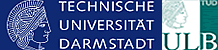Schnellsuche zur Expertensuche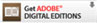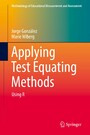Großes Bild

Applying Test Equating Methods - Using R
von: Jorge González, Marie Wiberg
Springer-Verlag, 2017
ISBN: 9783319518244

 Format:  PDF geeignet für: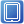Typ: B (paralleler Zugriff)KurzinformationThis book describes how to use test equating methods in practice. The non-commercial software R is used throughout the book to illustrate how to perform different equating methods when scores data are collected under different data collection designs, such as equivalent groups design, single group design, counterbalanced design and non equivalent groups with anchor test design. The R packages equate, kequate and SNSequate, among others, are used to practically illustrate the different methods, while simulated and real data sets illustrate how the methods are conducted with the program R. The book covers traditional equating methods including, mean and linear equating, frequency estimation equating and chain equating, as well as modern equating methods such as kernel equating, local equating and combinations of these. It also offers chapters on observed and true score item response theory equating and discusses recent developments within the equating field. More specifically it covers the issue of including covariates within the equating process, the use of different kernels and ways of selecting bandwidths in kernel equating, and the Bayesian nonparametric estimation of equating functions. It also illustrates how to evaluate equating in practice using simulation and different equating specific measures such as the standard error of equating, percent relative error, different that matters and others.nach oben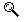Mehr zum Inhalt
 Kapitelübersicht Kurzinformation Inhaltsverzeichnis Leseprobe Blick ins Buch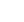NavigationInfo
© 2008-2022 ciando GmbH | Impressum | Kontakt | F.A.Q. | Datenschutz